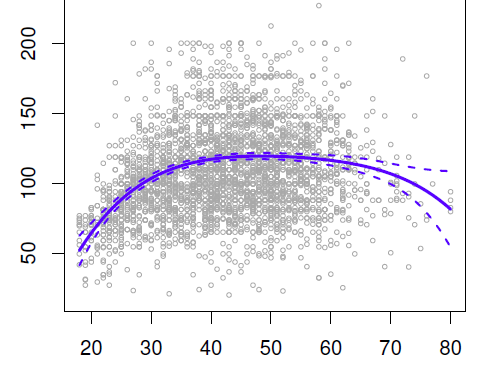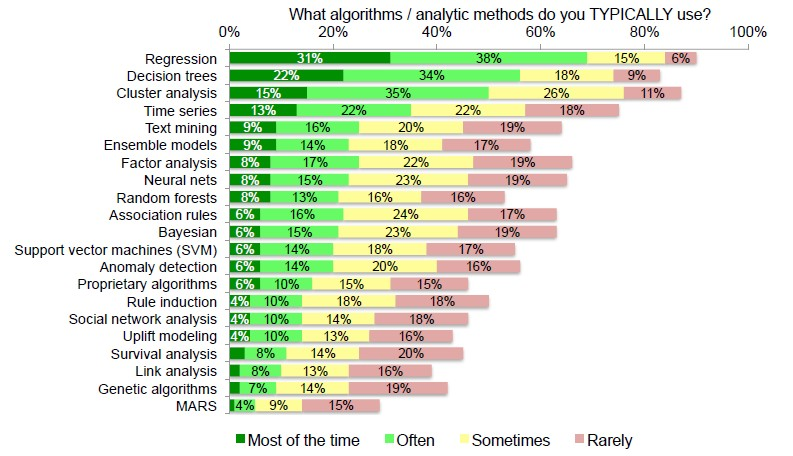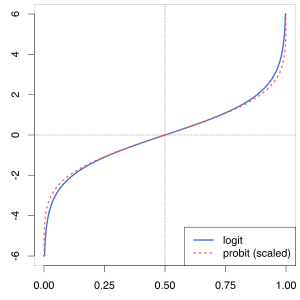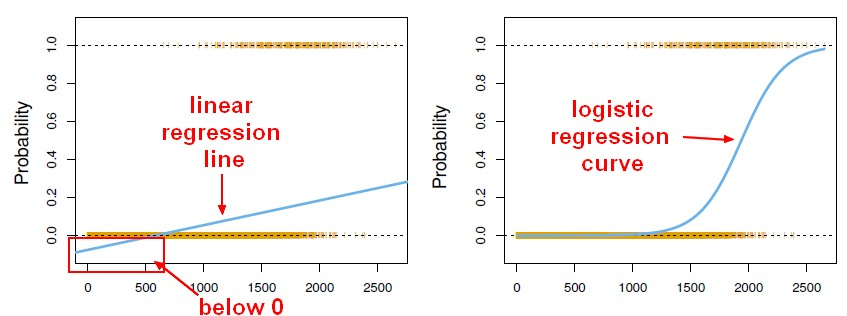# Machine Learning - Logistic regression (Classification Algorithm)

The prediction from a logistic regression model can be interpreted as the probability that the label is 1.

linear regression can also be used to perform classification problem.

Just by transforming the categorical target with continuous values.

The idea of logistic regression is to make linear regression produce probabilities. It's always best to predict class probabilities instead of predicting classes.

Logistic regression estimate class probabilities directly using the logit transform.

The Linear regression calculate a linear function and then a threshold in order to classify.

The result is logistic regression, a popular classification technique.

Logistic regression can be framed as minimizing a convex function but has no closed-form solution.

## Outcome

### Binary

For a two binary classification problem

• False = 0
• True = 1

If the predicted score $\hat{Y}$ > 0.5 then True.

In this case of a binary outcome, linear regression does a good job as a classifier, and is equivalent to linear discriminant analysis

See:

There's even some theoretical justification.

• A regression is an estimation of the conditional mean of Y given X.
• Therefore, the conditional mean of the 0, 1 variable given X is simply the probability that Y is 1 given X just by simple probability theory.

$$E(Y |X = x) = Pr(Y = 1|X = x)$$

### Multi-class

With a response variable with three possible values, we may choose this coding:

• Blue=1
• Green=2
• Red=3

This coding suggests an ordering, and in fact implies that the difference (distance) between blue and green is the same as between green and red.

Linear regression is then not appropriate. Multiclass Logistic Regression or Discriminant Analysis are more appropriate.

We can't perform a regression for each class (like multi‐response regression) because probabilities won’t sum to 1. It can be done as a joint optimization problem.

When the classes are well separated, it turns out that the parameter estimates for logistic regression are surprisingly unstable.

In fact, if you've go a feature that separates the classes perfectly, the coefficients go off to infinity.

Logistic aggression was developed in largely the biological and medical fields where you never found such strong predictors.

Now, you can do things to make logistic regression better behave. But it turns out linear discriminant analysis doesn't suffer from this problem and is better behaved in those situations.

Discover More(Machine learning|Inverse problems) - Regularization

Regularization refers to a process of introducing additional information in order to: solve an ill-posed problem or to prevent overfitting. This information is usually of the form of a penalty...Data Mining - (Discriminative|conditional) models

Discriminative models, also called conditional models, are a class of models used in machine learning for modeling the dependence of an unobserved variable y on an observed variable x. Discriminative...Data Mining - (Global) Polynomial Regression (Degree)

polynomials regression Although polynomials are easy to think of, splines are much better behaved and more local. With polynomial regression, you create new variables that are just transformations...Data Mining - Algorithms

An is a mathematical procedure for solving a specific kind of problem. For some data mining functions, you can choose among several algorithms. Algorithm Function Type Description Decision...Data Mining - Decision boundary Visualization

Classifiers create boundaries in instance space. Different classifiers have different biases. You can explore them by visualizing the classification boundaries. Logistic Regression method produces...Data Mining - Elastic Net Model

In statistics and, in particular, in the fitting of linear or logistic regression models, the elastic net is a regularized regression method that linearly combines the L1 and L2 penalties of the lasso...Data Mining - Probit Regression (probability on binary problem)

Probit_modelprobit model (probability + unit) is a type of regression where the dependent variable can only take two values. As the Probit function is really similar to the logit function, the probit...Loss functions (Incorrect predictions penalty)

Loss functions define how to penalize incorrect predictions. The optimization problems associated with various linear classifiers are defined as minimizing the loss on training points (sometime along with...Machine Learning - (Univariate|Simple) Logistic regression

A Simple Logistic regression is a Logistic regression with only one parameters. For the generalization (ie with more than one parameter), see Logistic regression comes from the fact that linear regression...R - K-fold cross-validation (with Leave-one-out)

Cross-validation in R. Leave-one-out cross-validation in R. Each time, Leave-one-out cross-validation (LOOV) leaves out one observation, produces a fit on all the other data, and then makes a...